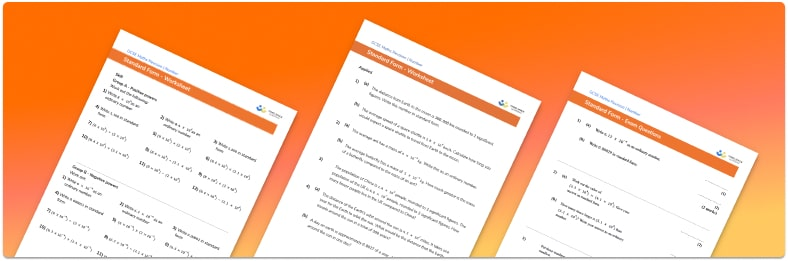# Standard Form Worksheet• Section 1 of the Standard Form worksheet contains 12 skills-based Standard Form questions, in 3 groups to support differentiation
• Section 2 contains 4 applied Standard Form questions with a mix of worded problems and deeper problem solving questions
• Section 3 contains 4 foundation and higher level GCSE exam style Standard Form questions
• Answers and a mark scheme for all Standard Form questions
• Follows variation theory with plenty of opportunities for students to work independently at their own level
• All questions created by fully qualified expert secondary maths teachers
• Suitable for GCSE maths revision for AQA, OCR and Edexcel exam boards

• This field is for validation purposes and should be left unchanged.

You can unsubscribe at any time (each email we send will contain an easy way to unsubscribe). To find out more about how we use your data, see our privacy policy.

### Standard form at a glance

Standard form, or standard index form, is a simple way of writing very large numbers or very small numbers. To write a number in standard form, we write the number as a decimal between 1 and 10, multiplied by a power of 10.  We call numbers that are not written in standard form ordinary numbers.

When converting numbers to standard form, we write any significant figures as a decimal between 1 and 10. We then need to maintain the place value of the number by multiplying the decimal by a power of 10. For example let’s look at the number 43000. The significant figures here are 4 and 3 so the decimal is 4.3. To maintain the place value of the number, we need to multiply 4.3 by 10000 or 104. Therefore 43000 can be written as 4.3×104. For very small numbers, the power of 10 will be negative.

We can convert standard form numbers back to ordinary numbers by multiplying the decimal by the power of 10. We can multiply and divide numbers written in standard form directly but to add or subtract numbers written in standard form it is best to convert them back to ordinary numbers.

Looking forward, students can then progress to additional number worksheets, for example aor aFor more teaching and learning support on Number our GCSE maths lessons provide step by step support for all GCSE maths concepts.

## Do you have KS4 students who need more focused attention to succeed at GCSE?There will be students in your class who require individual attention to help them succeed in their maths GCSEs. In a class of 30, it’s not always easy to provide.

Help your students feel confident with exam-style questions and the strategies they’ll need to answer them correctly with our dedicated GCSE maths revision programme.

Lessons are selected to provide support where each student needs it most, and specially-trained GCSE maths tutors adapt the pitch and pace of each lesson. This ensures a personalised revision programme that raises grades and boosts confidence.

Find out more